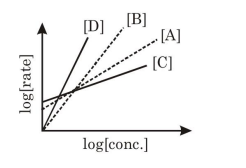# Consider the following reactions :

Question:

Consider the following reactions :

$\mathrm{A} \rightarrow \mathrm{P} 1 ; \mathrm{B} \rightarrow \mathrm{P} 2 ; \mathrm{C} \rightarrow \mathrm{P} 3 ; \mathrm{D} \rightarrow \mathrm{P} 4$

The order of the above reactions are $a, b, c$, and d, respectively. The following graph is obtained when $\log$ [rate] vs. $\log [$ conc $]$ are plotted:Among the following, the correct sequence for the order of the reactions is:

1. $\mathrm{a}>\mathrm{b}>\mathrm{c}>\mathrm{d}$

2. $\mathrm{c}>\mathrm{a}>\mathrm{b}>\mathrm{d}$

3. $\mathrm{d}>\mathrm{b}>\mathrm{a}>\mathrm{c}$

4. $\mathrm{d}>\mathrm{a}>\mathrm{b}>\mathrm{c}$

Correct Option: , 3

Solution:

(3) $\mathrm{d}>\mathrm{b}>\mathrm{a}>\mathrm{c}$